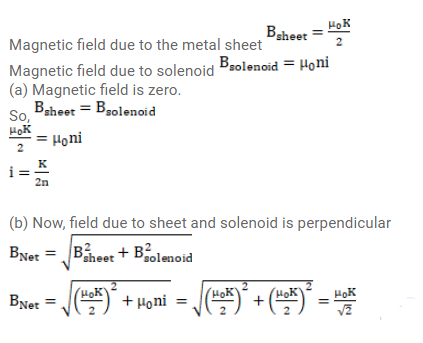# A tightly-wound, long solenoid is kept with its axis parallel to

Question:

A tightly-wound, long solenoid is kept with its axis parallel to a large metal sheet carrying a surface current. The surface current through a width dl of the sheet is $\mathrm{Kdl}$ and the number of turns per unit length of the solenoid is $\mathrm{n}$. The magnetic field near the center of the solenoid is found to bero.

(a) Find the current in the solenoid.

(b) If the solenoid is rotated to make its axis perpendicular to the metal sheet, what would be the magnitude of the magnetic field near its center?

Solution: